Luminescent properties of thermally activated delayed fluorescence molecule with intramolecular ππ interaction between donor and acceptor
Cai Lei, Fan Jianzhong, Kong Xiangpeng, Lin Lili, Wang Chuan-Kui
Shandong Province Key Laboratory of Medical Physics and Image Processing Technology, School of Physics and Electronics, Shandong Normal University, Ji’nan 250014, China

† Corresponding author. E-mail: linll@sdnu.edu.cn ckwang@sdnu.edu.cn

Project supported by the National Natural Science Foundation of China (Grant Nos. 11374195 and 21403133), Taishan Scholar Project of Shandong Normal University, China, the Promotive Research Fund for Excellent Young and Middle-aged Scientists of Shandong Province, China (Grant No. BS2014cl001), and the China Postdoctoral Science Foundation (Grant No. 2014M560571).

Abstract

Influence of intramolecular ππ interaction on the luminescent properties of thermally activated delayed fluorescence (TADF) molecule (3, 5-bis(3,6-di-tert-butyl-9H-carbazol-9-yl)-phenyl)(pyridin-4-yl) methanone (DTCBPY) is theoretically studied by using the density functional theory (DFT) and time-dependent density functional theory (TD-DFT). Four conformations (named as A, B, C, and D) of the DTCBPY can be found by relax scanning, and the configuration C corresponds to the luminescent molecule detected experimentally. Besides, we calculate the proportion of each conformation by Boltzmann distribution, high configuration ratios (44% and 52%) can be found for C and D. Moreover, C and D are found to exist with an intramolecular ππ interaction between one donor and the acceptor; the intramolecular interaction brings a smaller Huang–Rhys factor and reduced reorganization energy. Our work presents a rational explanation for the experimental results and demonstrates the importance of the intramolecular ππ interaction to the photophysical properties of TADF molecules.

PACS: 85.60.Bt
1. Introduction

Recently, a molecule named (3,5-bis(3,6-di-tert-butyl-9H-carbazol-9-yl)-phenyl) (pyridin-4-yl) methanone (DTCBPY) (as shown in Fig. 1) was synthesized and the light-emitting properties were studied in solvent and solid states. It was found that the intramolecular interaction between the donor and adjacent acceptor units plays an important role in displaying efficient TADF properties. In this paper, the DTCBPY molecule is chosen as a model system to study the influence of the intramolecular interaction between donor groups and acceptors on its photophysical properties. First-principles calculations combined with the excited states dynamics will provide more information on the relationship between the intramolecular interaction and the photophysical properties.

 Figure Option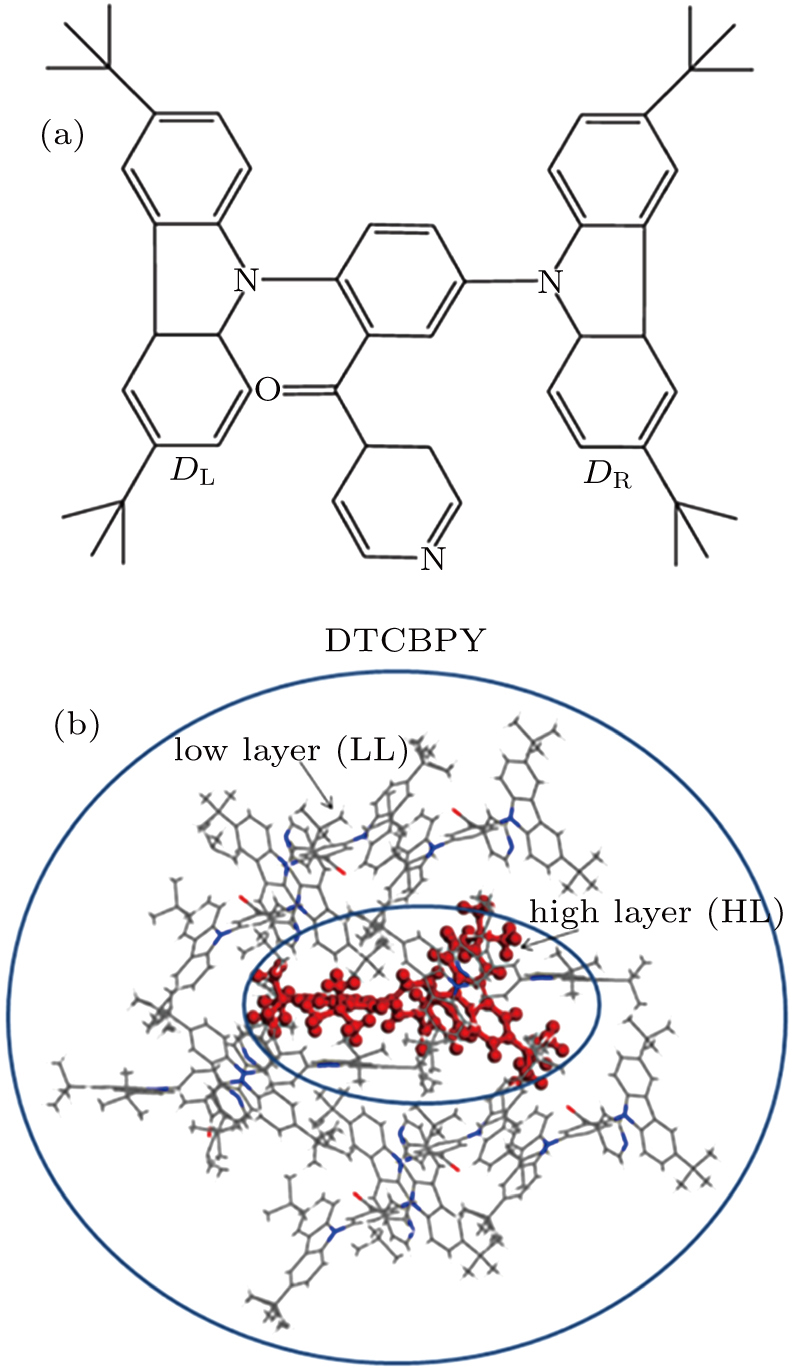Fig. 1. (color online) (a) Geometry structure of the studied molecule DTCBPY. (b) ONIOM model: the centered C configuration is treated as a high layer and the surrounding configurations are fixed as a low layer.
2. Computational methods

In this paper, the density functional theory (DFT) is used to perform the optimization and electronic structure calculation of the molecule in ground state. The time-dependent density functional theory (TD-DFT) is employed to investigate the properties of the excited states. In the calculations, the pbe0-1/3 exchange functional and the 6-31G(d) basis set are used. The polarizable continuum model (PCM) is used to take into account the solvent effect in all simulations.[24,25]

The photoluminescence quantum yield (PLQY) is determined by the radiative rate (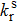) and the non-radiative rate (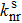).can be computed using the Einstein spontaneous emission rate equation, which is written asHere f is the oscillator strength without dimension and ΔEfi in units of wavenumber (cm−1) is the energy difference between the initial state and the final state.reflects the energy relaxation process through molecular vibration, and it has a close relationship with the Huang–Rhys (HR) factor and reorganization energy (λ). The HR and λ can be calculated with the DUSHIN module in the MOMAP (Molecular Materials Property Prediction Package) program, which shows superiority in describing and predicting the optical properties of polyatomic molecules.[27,28]

In order to visualize the intramolecular interaction between the donor and the acceptor, the reduced density gradient (RDG) method is adopted. The RDG formula is written as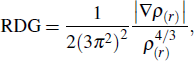where ρ(r) is the electronic density. Through analysis of the RDG values, one can easily distinguish the area of near nuclei, near chemical bonds region, weak interacting regions and the edges of the molecule. This method can also highlight the areas of ππ intramolecular interaction in the system and help to visualize the region that is associated with the intramolecular ππ interaction.

In experiment, the PLQY of the molecule in toluene is 30.3%, while it reaches up to 91.4% in solid state. It means that the luminescence efficiency of the molecule is greatly enhanced in the aggregation state. To explain this phenomenon, the combined quantum mechanics and molecular mechanics (QM/MM) method is adopted to study the electroluminescent properties of the DTCBPY molecule by considering the environment effect in aggregation state. The initial structure is obtained from the x-ray crystal structure detected experimentally. The QM/MM calculation is realized with the ONIOM method in Gaussian 09 program. In this model, two “layers” are constructed, as shown in Fig. 1(b). The central molecule is treated as a high layer and calculated by the quantum mechanical method. The surrounding molecules are treated as a low layer and computed by molecular mechanics with UFF forces field.

3. Results and discussion
3.1. Molecular geometric structure

The DTCBPY molecule consists of two donors and one acceptor, which possesses a flexible configuration, as shown in Fig. 1(a). By relax scanning, we find that the molecule has four configurations as shown in Fig. 2 (with the short names of A, B, C and D). For A and C, the molecular surfaces of the two donor groups are perpendicular to each other, while the surfaces of the two donor groups in B and D are parallel. For A and B, the surface of the acceptor group is almost perpendicular to the adjacent donor group. While, for C and D, the dihedral angle between the two groups is almost 45°. The population proportion of each configuration in the ground state is calculated according to the Boltzmann distribution which can be written as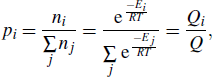where p is the proportion, i represents the configuration, ni is the number of molecules in the i-th configuration, and E is the energy of the configuration. T is the temperature (in units of K), and R is the ideal gas constant. Q is the partition function. By using the relative energies of different conformations, the Boltzmann distribution formula can be rewritten asHere ΔEi is the relative value of conformation i, and the values are shown in Table 2. The proportions of the four conformations calculated based on the above formula are also listed in Table 2. In the table, the energy of D is set as the benchmark. The energy of C is only 0.1 kcal/mol larger than C, while A and B are 2.08 kcal/mol and 1.91 kcal/mol larger than D respectively. The population proportions of C and D are 44% and 52% respectively, while the proportions of A and B are 1.5% and 2.5%. The potential energy surface of the molecule in ground state is shown in Fig. 3. From the figure, we can see that the energy barrier from C to D is only 0.05 eV. The backward energy barrier is also quite small (0.06 eV). Consequently, we conclude that configurations C and D are easily transformed to each other. However, the energy barrier from C to B is 0.12 eV, which means that it is impossible for configuration C to transform to B. While the energy barrier from B to C is only 0.03 eV, and the energy barriers between A and B (forward and backward) are all quite small (0.36 eV). Based on the values above, we can conclude that most of the molecules are in configurations C and D. The absorption and emission wavelengths for different configurations are shown in Table 1. We can see that the absorption wavelengths for A and B (428 nm and 435 nm) are a little longer than those of C and D (408 nm and 410 nm). All the values are close to the experimental values (418 nm). The emission wavelengths for A and B (590 nm and 600 nm) are about 70 nm longer than those of C and D (519 nm and 527 nm). In comparison with the experimental value (508 nm), we conclude that most possible configurations of the molecule are C and D. The result is consistent with the energy analysis above. In addition, we find that the configuration C agrees with the molecular structure detected by the x-ray diffraction, which also confirms our conclusion.

 Figure Option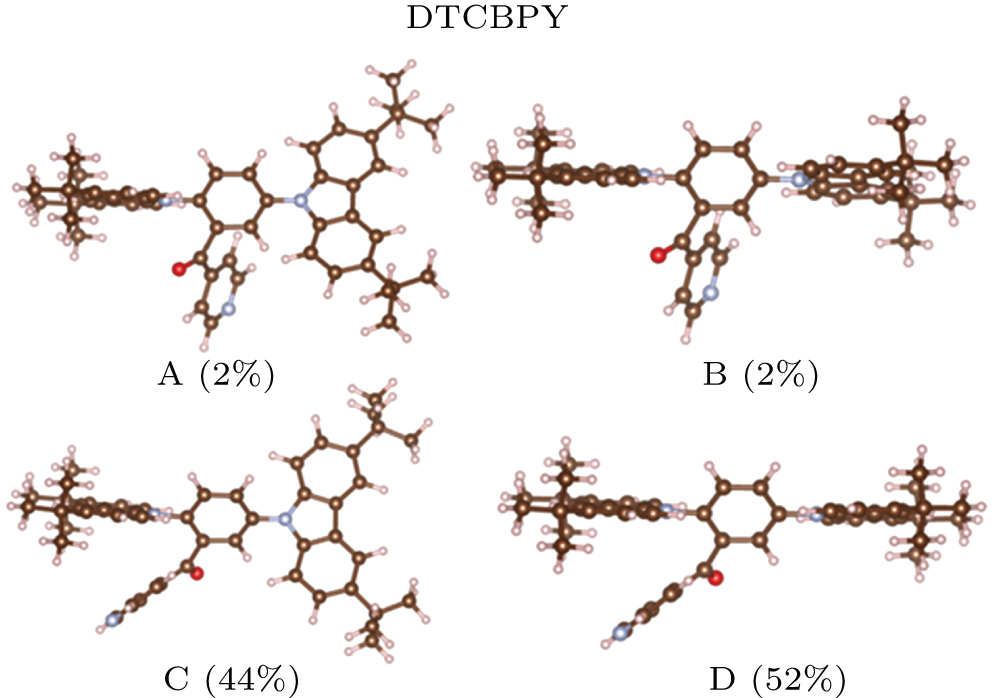Fig. 2. (color online) Four configurations of the DTCBPY and the proportion ratios of the configurations.
 Figure OptionFig. 3. (color online) Potential energy surface and energy barrier of the molecule. The geometric structure of the molecule in each point is also presented.Table 1.

Absorption wavelength, emission wavelength, oscillator strength, radiative rate, stokes shift (λshift), and energy gap for the four configurations.

.Table 2.

Relative energy of the four conformations and their proportions.

.

Based the analysis above, the configurations of C and D have the most possible configurations. From the configurations of C and D, we can see that the acceptor group is close to one donor group, thus we deduce that there is intramolecular ππ interaction between them. To visualize the intramolecular interaction more intuitively, the RDG method is used (shown in Fig. 4). From this figure, we can see that there is significant interaction between the acceptor and the adjacent donor group. This confirms that there is intramolecular ππ interaction between them.

 Figure OptionFig. 4. (color online) Intramolecular π–π interaction between the acceptor and the donor nearby described by the RDG method. The weak interacting area is marked with the red circle.
3.2. Excited state property and energy gap

The electron distributions of the highest occupied molecular orbital (HOMO) and the lowest unoccupied molecular orbital (LUMO) as well as their energy levels for four configurations are shown in Fig. 5. The energies of HOMOs for A, B, C, and D are −5.86 eV, −5.84 eV, −5.89 eV, and −5.87 eV, respectively, and they are almost equal. While the energies of LUMOs for C and D are −1.82 eV and −1.80 eV, respectively, which are larger than those of A and B (−1.92 eV and −1.91 eV). Based on the analysis of the frontier molecular orbitals, we find that the position of the acceptor groups can influence the energy levels of LUMOs. In comparison with A and B, we can conclude that the ππ interaction between the donors and acceptors can increase the energy levels of LUMOs. In addition, one can see that the electron distributions of HOMOs and LUMOs for A and B are also a little different from those of C and D. The overlap between HOMOs and LUMOs for C and D (0.274 and 0.277) is larger than that for A and B (0.212 and 0.213), which can induce a different radiation rate () and ΔEST. The oscillator strengths (f), radiation rates, and ΔEST for the four configurations are shown in Table 1. The oscillator strengths of A and B are smaller than those of C and D. The radiation rates for A and B are 1.03 × 106 s−1 and 1.3 × 106 s−1, respectively, while they are 3.6 × 106 s−1 and 3.4 × 106 s−1 for C and D. The kr of A and B are also far smaller than those of C and D. The corresponding ΔEST for A and B are 0.18 eV and 0.22 eV, respectively. They are increased to 0.30 eV and 0.32 eV for C and D. From these results, we find that larger overlap between HOMOs and LUMOs will induce increased radiation rate () and smaller ΔEST. This can be explained using the formula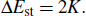Figure Option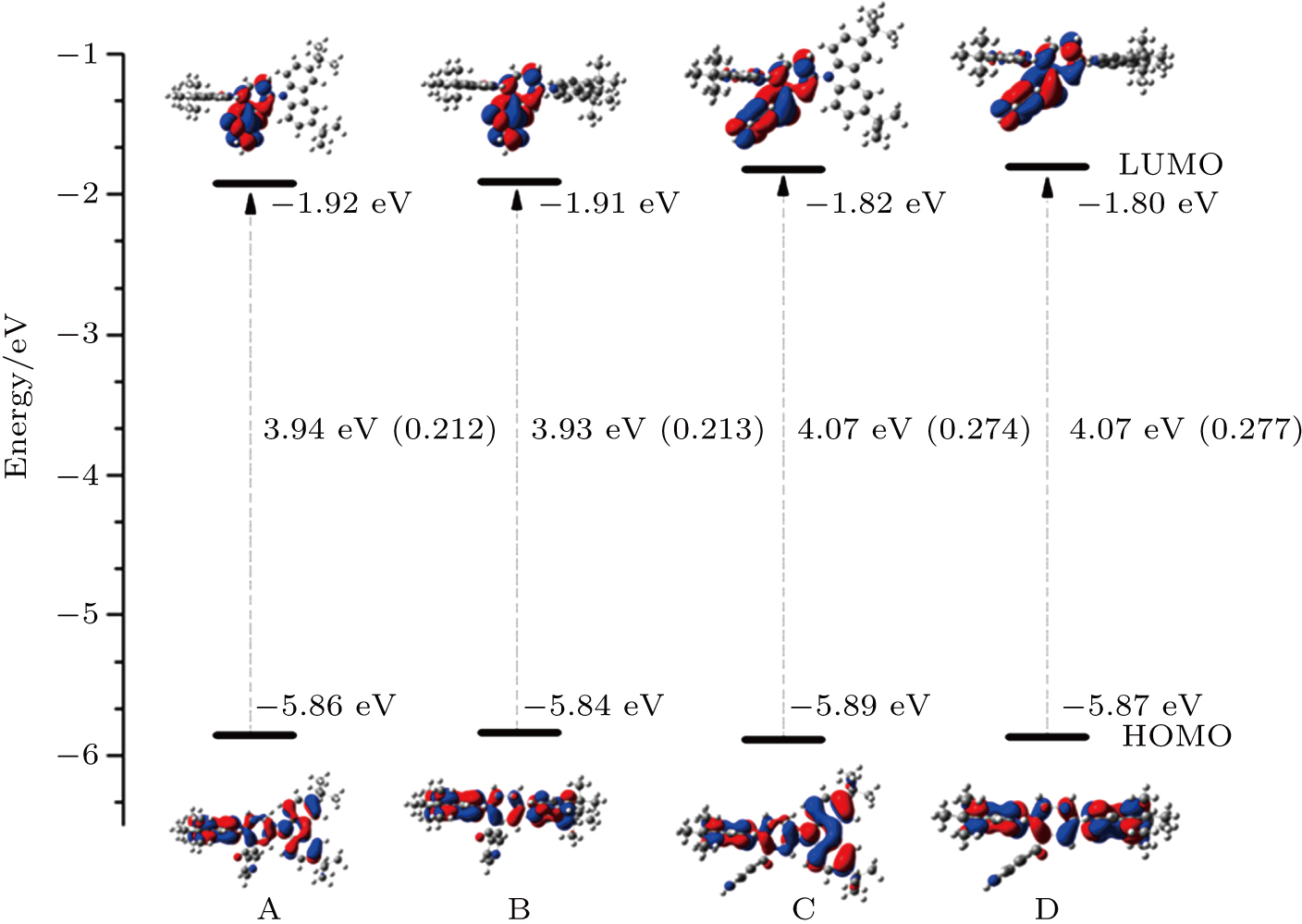Fig. 5. (color online) Distributions of frontier molecular orbitals of the configurations. The energy levels of HOMOs and LUMOs as well as their energy gaps are also shown. The overlaps between HOMOs and LUMOs are listed in the bracket.

Here K is the exchange energy, and it can be calculated by the formulawhere ϕH and ϕL represent the wave functions of the HOMO and LUMO, respectively. For TADF molecules, an endothermic up-conversion process from T1 to S1 state can be thermally activated when ΔEst is small. The small ΔEst can be achieved by decreasing the overlap between HOMOs and LUMOs. The f is determined by the transition dipole moment μ which can be written as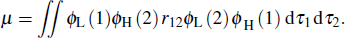One can see that the overlap between HOMO and LUMO can also influence μ. Thus f will also be determined by the HOMO–LUMO overlap. The larger the overlap, the bigger the f. As a result, the TADF molecules should have a proper overlap between HOMOs and LUMOs, which can be regulated by adjusting the intramolecular interaction. Based on the analysis above, we find that the intramolecular ππ interaction between the acceptor and the donor can effectively enhance the light-emitting efficiency, which may help us to design highefficient light-emitting materials.

3.3. Influence on the non-radiative rate

Reorganization energy (λ) can be expressed as a summation of the contributions from normal mode (NM) relaxation in the harmonic oscillator approximation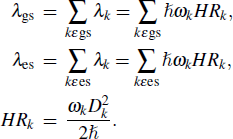In the formula, HRk represents the Huang–Rhys factor for the k-th mode, and Dk is the displacement for mode k between the equilibrium geometries of S0 and S1. The maximum Huang–Rhys factor (HRmax) and the corresponding λ are listed in Table 3. It is found that the HRmax values for A and B are 16.8 and 23.2, while they are 9.3 and 8.9 for C and D. The λ values for A and B are 4869 meV and 7492 meV, which are much larger than those for C and D (1165 meV and 1175 meV). As the non-radiative rate is almost in proportion with the reorganization energy, we can deduce a smaller non-radiative rate for C and D. It is also indicated that the intramolecular ππ interaction may induce small HR and λ, and in turn result in a smalland a high PLQY. By detailed analysis of the vibration mode with the largest Huang–Rhys factor, we find that it corresponds to the out-of-face vibration of the acceptor group and the donor groups. This kind of motion may induce the change of the dihedral angle between the acceptor and the donor. Furthermore, the intramolecular ππ interaction will be influenced.Table 3.

Reorganization energy λ and the maximum Huang–Rhys factor HRmax for four configurations.

.

Further, the QM/MM method is used to study the change of the dihedral angles of the molecule in ground state and excited state in solution and in solid state (as listed in Table 4). In toluene, the dihedral angle between the surfaces of the left donor (DL) and the acceptor in ground state is 57.79°, and it is 51.03° for the right donor (DR) and the acceptor. For the S1 state, they become 50.77° and 45.89°. The dihedral angle changes between the ground state and the S1 is 7.02° and 5.14°, respectively. In solid state (see Fig. 1), the dihedral angles are 61.2° and 57.0° in the ground state, and they are 61.3° and 54.6° in S1. The dihedral angle changes are only 0.1° and 2.4°, respectively. Compared with the dihedral angle change in a solvent, the dihedral angle change in a solid decreases obviously. The geometric change is suppressed in a solid, which will induce a smaller reorganization energy, and decrease the. As a result, the PLQY will be enhanced. In experiment, the PLQY in a solid is greater than that in a solvent, which confirms our conclusion. Based on our results, we deduce that the molecule has the characteristics of aggregation induced enhanced emission.Table 4.

Dihedral angles between the left (right) donor and the acceptor (DL and DR respectively) for the molecule in ground state and S1 state. The differences are also shown.

.
4. Conclusion

We have studied a TADF molecule named DTCBPY with the DFT and TD-DFT methods. Through relax scanning, we find that the molecule has four different conformations, and the configuration C is consistent with the molecule structure detected in the experiment. Our theoretical results also indicate that both C and D have the most possible configurations for DTCBPY. By using the RDG function, we confirm that there is significant ππ interaction between the acceptor and the donor groups nearby in C and D. By analysis of the Huang–Rhys factor and the reorganization energy, we deduce that the intramolecular interaction can induce a smaller non-radiative rate and a high PLQY. In addition, the QM/MM method is used to study the structural changes of the molecule between the ground state and the S1 state in the solid state. Compared with the dihedral angle change in a solvent, the dihedral angle change in a solid state decreases obviously, which may result in suppressed non-radiative transition and enhanced luminous efficiency. Based on our calculation results, we deduce that the molecule may have the properties of aggregation induced enhanced emission. Our theoretical research provides valuable information for the design of highly-efficient organic light-emitting molecules.

Reference
  Baldo M A Thompson M E Forrest S R 2000 Nature 403 750  Sun Y Giebink N C Kanno H Ma B Thompson M E Forrest S R 2006 Nature 440 908  Wang Z J Zhao J Zhou C Qi Y G Yu J S 2017 Chin. Phys. 26 047302  Nie H Zhang B Tang X Z 2007 Chin. Phys. 16 730  Cui L S Deng Y L Tsang D P K Jiang Z Q Zhang Q Liao L S Adachi C 2016 Adv. Mater. 28 7620  Lee S Y Yasuda T Komiyama H Lee J Adachi C 2016 Adv. Mater. 28 4019  Duan C B Li J Han C M Ding D X Yang H Wei Y Xu H 2016 Chem. Mater. 28 5667  Fan J Z Lin L L Wang C K 2016 Chem. Phys. Lett. 652 16  Chen Z Liang X Zhang H Y Xie H Liu J W Xu Y F Zhu W P Wang Y Wang X Tan S Y Kuang D Qian X H 2010 J. Med. Chem. 53 2589  Lin L L Wang Z J Fan J Z Wang C K 2017 Org. Electron. 41 17  Nasu K Nakagawa T Nomura H Lin C J Cheng C H Tseng M R Yasuda T Adachi C 2013 Chem. Commun. (Camb) 49 10385  Zhang Q S Li J Shizu K Huang S P Hirata S Miyazaki H Adachi C 2012 J. Am. Chem. Soc. 134 14706  Zhang Q S Li B Huang S P Nomura H Tanaka H Adachi C 2014 Nat Photon. 8 326  Lee D R Choi J M Lee C W Lee J Y 2016 ACS Appl. Mater. Interfaces 8 23190  Dos Santos P L Ward J S Bryce M R Monkman A P 2016 J. Phys. Chem. Lett. 7 3341  Fan J Z Lin L L Wang C K 2017 J. Mater. Chem. C accepted  Mei J Leung N L Kwok R T Lam J W Tang B Z 2015 Chem. Rev. 115 11718  Zhang T Peng Q Quan C Y Nie H Niu Y L Xie Y J Zhao Z J Tang B Z Shuai Z G 2016 Chem. Sci. 7 5573  Luo J D Xie Z L Lam J W Y Cheng L Chen H Y Qiu C F Kwok H S Zhan X W Liu Y Q Zhu D B Tang B Z 2001 Chem. Commun. 1740  Peng Q Fan D Duan R H Yi Y P Niu Y L Wang D Shuai Z G 2017 J. Phys. Chem. 121 13448  Zhang Y G Zhang D D Cai M H Li Y L Zhang D Q Qiu Y Duan L 2016 Nanotechnology 27 094001  Tsujimoto H Ha D G Markopoulos G Chae H S Baldo M A Swager T M 2017 J. Am. Chem. Soc. 139 4894  Rajamalli P Senthilkumar N Gandeepan P Huang P Y Huang M J Zheng C Wu R Yang C Y Chiu M J Chu L K Lin H W Cheng C H 2016 J. Am. Chem. Soc. 138 628  GAUSSIAN 09, references in http://www.gaussian.com/  Zhang Y J Zhang Q Y Ding H J Song X N Wang C K 2015 Chin. Phys. 24 023301  Peng Q Niu Y L Shi Q H Gao X Shuai Z G 2013 J. Chem. Theory Comput. 9 1132  Zhang T Jiang Y Q Niu Y L Wang D Peng Q Shuai Z G 2014 J. Phys. Chem. 118 9094  Reimers J R 2001 J. Chem. Phys. 115 9103  Johnson E R Keinan S Mori-Sanchez P Contreras-Garcia J Cohen A J Yang W T 2010 J. Am. Chem. Soc. 132 6498  Fan J Z Cai L Lin L L Wang C K 2016 J. Phys. Chem. A 120 9422  Lu T Manzetti S 2014 Struct. Chem. 25 1521  Tao Y Yuan K Chen T Xu P Li H H Chen R F Zheng C Zhang L Huang W 2014 Adv. Mater. 26 7931